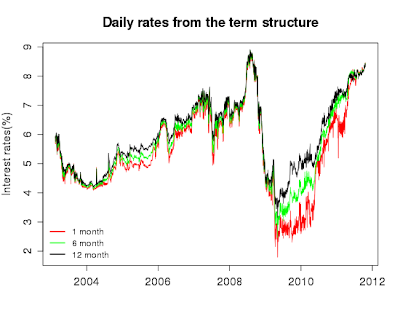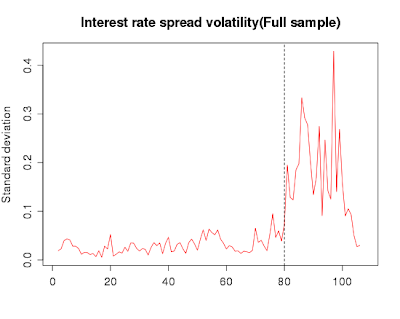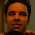## Friday, 1 June 2012

### Term structure of interest rate spread volatility : Unit root test

Recently, I was working on my master's thesis and came across an interesting observation regarding the term structure of interest rate spread volatility that I wish to share. Let me first try and throw some light on the jargon that I have used. To begin with, term structure of interest rates is basically a curve plotting the market expectation of nominal interest for different maturities. How do we get this term structure? Well, the trading of bonds (i.e fixed income securities) happen in the secondary market for debt instruments. Since, bonds have fixed coupon payments at fixed period of time, we can actually look at combinations of discount rates for these different (but fixed) time periods and discount these future coupon payments by their respective discount rates to arrive at the current price, i.e the one discovered in the market (simple application of the present value principal). Now the beauty is that we have a number of securities trading in the debt market, each with different maturity and coupon payment. So the discovered price of all these securities can be used to fit a function to the rate at which different coupon payments have been discounted, this results in what we called the term structure of interest rates or sometimes referred to as zero coupon yield curve (zcyc). The most popular functional form adopted by applied researchers, and also the one adopted by the Clearing Corporation of India ltd (CCIL) is the Nelson-Siegel-Svensson (NSS) equation. But I shall not delve into that in detail. My observation pertains to the dynamics of the volatility of the term structure of interest rate spread in India.

Let me start by plotting the daily interest rates from the term structure starting February 2003.Daily term structure rates
Next we simply plot the monthly standard deviation of the interest rates by at different maturities.

Things are straightforward till now but when I plot the standard deviation of the interest rate spread things get a little strange. Well, to start with we know that spread is already a first differenced variable, in the sense that it is basically the difference between interest rate at 2 different maturities. So, apriori we would not expect it to be a non-stationary series. Let us examine how the plot of the interest rate spread volatility looks like. For simplicity and sake of exposition we plot only the (3-1) month spread volatility.(3-1) month interest rate spread volatility

Now this series turns out to be non-stationary when passed through the adf.test(). This counter-intuitive observation was difficult for me to digest so I delved into the problem to figure out what could possibly be going wrong. To start with my sample size was not very large (~90 obs) which could lead to the wrong rejection of the null of stationarity. Secondly, there is a structural change to be suspected in the series approximately near September 09. Now keeping this in mind when we ran the adf.test() for the sample before Sept'09 and after Sept'09, surprisingly,  we find that both the samples are non-stationary. Looking at the plot one would not suspect such a result. From a casual visual inspection it seems that the series is stationary before Sept'09 but could be non-stationary post Sept'09. So to solve this puzzle, we break the sample into pre-Sept'09 and post Sept'09 and do some simple visualizations.(3-1) month Interest rate spread volatility for the entire sample
Now if we look at the adf.test() results the high p-value suggests that the series, both pre and post the break, is no where near to being an I(0) series.Interest rate spread volatility for the split sample
When we visualize the series pre and post break the intuition becomes clear as the movement of the series appear to be quite random and the constant mean property of a stationary series seems to be violated (Refer to this previous post for a similar discussion). The reason why a split sample visualization made more sense here was because the movements post Sept'09 were so large that the actual dynamics (which are actually quite random) were not visible in the same plot, thus giving the false impression of stationarity in the pre break period. However, this simple split sample visualization makes the results seem quite intuitive.

1.Hi,
Nice post.
It is indeed counter intuitive to have such a result. Google "Choosing the Lag Length for the" and you will find that the adf.test() has a very low power against "close to stationarity" processes. Your result probably has something to do with the way the number of lags are chosen. Remember the yields are highly persistent. Apart from that, fact that we have monthly data renders relatively few obs, which also plays a p.value role.
My suggestion is to test it using to the kpss statistic, which makes more sense to me in this case.
Cheers
Eran

1.Hi Eran,

Thank you for your comment, and apologies for my late response as I have been travelling. As per your suggestion I did try the KPSS test and that yields the same result that the series is non-stationary. I believe the issue is because of small sample size, because when I work with daily rates the resultant series is stationary.

~
Shreyes

2.Thank you for sharing such great information.
It is informative, can you help me in finding out more detail on
house loan interest rates.

3.It was a wonderful article with proper demonstration with facts and figures.
Once Go through this aarticle..
Theories of term structure

4.Really it is very useful for us..... the information that you have shared is really useful for everyone.
I am also business study blogger . i have also some articles about Business Environment please visit once and give me some feedback. Thank You.